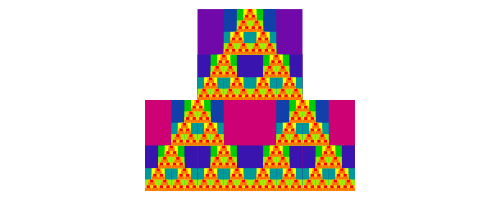Diagrams In Hakyll!

Posted on June 23, 2018 by Noon van der Silk

I stumbled across this blogpost of Corentin Dupont where he put together a library that allows you to modify your hakyll blog so that you can have inline diagrams! As anyone knows, this was amazingly exciting to me, because I love diagrams.

So I quickly tried to set it up; but, much to my sadness it didn’t immediately work.

Luckily, however, I was able to make it worked by hacking around in the two relevant repos:

The main result is a function, pandocCompilerDiagrams, that I included into my hakyll site file like so:

match "posts/*" $do route$ setExtension "html"
compile $(pandocCompilerDiagrams "images/diagrams" <|> pandocMathCompiler) >>= loadAndApplyTemplate "templates/post.html" postCtx >>= saveSnapshot "content" >>= loadAndApplyTemplate "templates/default.html" postCtx >>= relativizeUrls And so now, I can have inline diagrams! Check it out: Imagine we had a circle:example = circle 1 But now, what if the circle was repeated 5 timesexample = hcat (take 5$ repeat (circle 1))

Cool!

To celebrate, let’s draw the Sierpinksi triangle:

The basic building block:sierp d = d === (d ||| d) # centerXY
example = sierp (triangle 1)

Let’s go!sierp d = d === (d ||| d) # centerXY
example = foldl (\d _ -> sierp d) (triangle 1) [1..3]

Colours!import Data.Colour.Palette.ColorSet

color n = rybColor (n*2)

sierp d n = d1 === (d2 ||| d2) # centerX
where
d1 = d # bg (color n)
d2 = d # bg (color (n+1))

example = foldl step d0 [0..5]
where
d0       = triangle 1 # lw 0
step d n = sierp d (n*2)

Happy days!